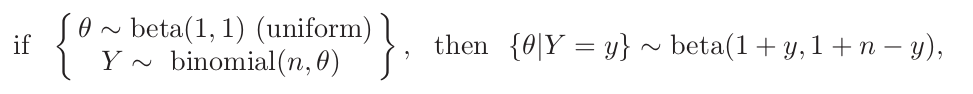# One Parameter Models

##### Posted on Jun 04, 20170 Comments
Tags: Bayesian Inference

## What is one-parameter model

A class of sampling distributions that is indexed by a single unknown parameter.

## The binomial model

### Happiness data

$n = 129$ individuals

If happiness, $Y_i = 1$, otherwise, $Y_i=0$.

### Data and posterior distribution

An important formula:

#### The beta distribution

Three properties:

1. $mode(\theta)=\frac{a-1}{a-1+b-1}$
2. $E(\theta)=\frac{a}{a+b}$
3. $Var(\theta)=\frac{E(\theta)E(1-\theta)}{a+b+1}$### Confidence regions

Frequentist coverage lacks a post-experimental interpretation.

### relationship

an interval that has 95% Bayesian coverage additionally has the property that

where $\vert \epsilon_n\vert<a/n$

### quantile-based interval

$n=10$ conditionally independent draws of a binary random variable.

using a uniform prior distribution for $\theta$

a = 1; b = 1 ## prior
n = 10; y =2 ## data
qbeta(c(.025, .975), a+y, b+n-y)
## 0.06021773 0.51775585


### Hightest posterior density region

There is a simple R code which can return the HPD region.

## The Poisson Model

Two properties

• $E(Y\mid\theta)=\theta$
• $Var(Y\mid \theta)=\theta$

### Gamma distribution

gamma(a,b)

• $E(\theta) = a/b$
• $Var(\theta) = a/b^2$
• $mode(\theta)=(a-1)/b (if\;a > 1\;or\; 0)$

## Exponential families and conjugate priors

A one-parameter exponential family model is any model whose densities can be expressed as

conjugate prior distribution

Published in categories Note